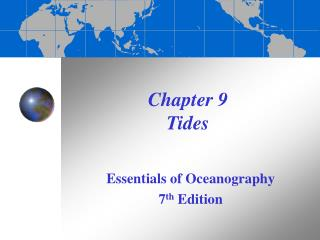DownloadDownload PresentationChapter 9 Tides

# Chapter 9 Tides

Télécharger la présentation## Chapter 9 Tides

- - - - - - - - - - - - - - - - - - - - - - - - - - - E N D - - - - - - - - - - - - - - - - - - - - - - - - - - -
##### Presentation Transcript

1. Chapter 9 Tides Essentials of Oceanography 7th Edition

2. What causes tides? • Tides are created by the imbalance between two forces: • Gravitational force of the Moon and Sun on Earth • If mass increases (), then gravitational force increases () • If distance increases (), then gravitational force greatly decreases () • Centripetal (center-seeking) force required to keep bodies in nearly circular orbits

3. Gravitational forces on Earth due to the Moon • Force decreases with increasing distance • Force is directed toward the Moon’s center of mass Figure 9-2

4. Centripetal forces on Earth due to the Moon • Force is the same everywhere on Earth • Force is directed perpendicular to Earth’s center everywhere on Earth Figure 9-3

5. Resultant forces • Resultant forces are: • The difference between gravitational (G) and centripetal (C) forces • Directed away from Moon on the side of Earth opposite Moon • Directed toward Moon on the side of Earth facing Moon Figure 9-4

6. Tide-generating forces • Tide-generating forces are the horizontal component of the resultant force • Maximized along a “latitude” of 45º relative to the “equator” between the zenith and nadir Figure 9-5

7. Tidal bulges • Tide-generating forces produce 2 bulges: • Away from Moon on side of Earth opposite Moon • Toward Moon on side of Earth facing Moon • Earth rotates into and out of tidal bulges, creating high and low tides Figure 9-6

8. The lunar day • Tidal bulges follow Moon as it rotates around Earth • Lunar day is 50 minutes longer than a solar day because the Moon is moving in its orbit around Earth Figure 9-7

9. Relative sizes and distances on Earth, Moon, and Sun • The Sun is much more massive than the Moon but much further away • Solar bulges are 46% the size of lunar bulges Figure 9-8

10. The monthly tidal cycle(29½ days) • About every 7 days, Earth alternates between: • Spring tide • Alignment of Earth-Moon-Sun system (syzygy) • Lunar and solar bulges constructively interfere • Large tidal range • Neap tide • Earth-Moon-Sun system at right angles (quadrature) • Lunar and solar bulges destructively interfere • Small tidal range

11. Effect of declination • The plane of the Moon’s orbit is tilted 5º with respect to the ecliptic • The center of the tidal bulges may be up to a maximum of 28.5º from the Equator Figure 9-11

12. Effect of elliptical orbits • Tidal ranges are greater when: • The Moon is at perigee • The Earth is at perihelion Figure 9-12

13. Predicted idealized tides Figure 9-13

14. Summary of tides on an idealized Earth • Most locations have two high tides and two low tides per lunar day • Neither the two high tides nor the two low tides are of the same height because of the declination of the Moon and the Sun • Yearly and monthly cycles of tidal range are related to the changing distances of the Moon and Sun from Earth • Each week, there would be alternating spring and neap tides

15. Tides in the ocean • Cotidal map shows tides rotate around amphidromic points • More realistic pattern of tides in the ocean Figure 9-14

16. Tidal patterns • Diurnal • One high and one low tide each (lunar) day • Semidiurnal • Two high and two low tides of about the same height daily • Mixed • Characteristics of both diurnal and semidiurnal with successive high and/or low tides having significantly different heights

17. Tidal patterns in the U.S. • Internet site showing tidal predictions for various U.S. locations Figure 9-15

18. Monthly tidal curves Figure 9-16

19. The Bay of Fundy: Site of the world’s largest tidal range • Tidal energy is focused by shape and shallowness of bay • Maximum spring tidal range in Minas Basin = 17 meters (56 feet) Figure 9-17

20. Coastal tidal currents • Tidal currents occur in some bays and rivers due to a change in tides • Ebb currents produced by outgoing tides • Flood currents produced by incoming tides Figure 9-18

21. Tidal bore = a true tidal wave • Wall of water that moves upriver • Caused by an incoming high tide • Occurs in some low-lying rivers • Can be large enough to surf or raft Figure 9B

22. Grunion and the tides • Grunion are the only fish that come completely out of water to spawn • Spawning cycles are timed precisely with the tides Figure 9C

23. End of Chapter 9 Essentials of Oceanography 7th Edition